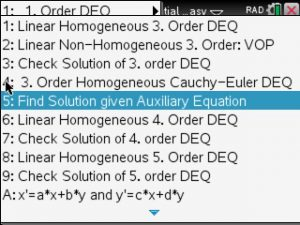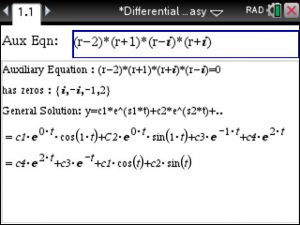## Solving Fractions and Quadratics using the TiNSpire – Step by Step

Say your teacher has some fancy fractions to solve for you and you have a volleyball game , play practice and to prepare for the SAT next Saturday. So you take out your TiNspire CX CAS, launch the STEP BY STEP EQUATION SOLVER app from www.TiNspireApps.com and get a quick lesson on how to solve those fractions…which turns out not too difficult after following the provided steps below:

We select option 5 :

1. problem:

to get 5/14 . The trick is to multiply the given fractions by the product of their denominators (bottoms) to get a much easier equation to solve.

Here is another one:

and

It always works!

Quadratic equations can also be solved step by step. Here is one:

and

Even equations containing only variables can be solved (for x):

Posted on Categories algebra, tinspirecx

## Auxiliary / Characteristic Equation to Solution of Differential Equation – Step by Step – using the TiNSpire CX

Auxiliary Equation Solution using the Tinspire CX

Finding the Solution given Auxiliary (also called Characteristic) Equation of a Differential Equation is a fun exercise using the Differential Equation Made Easy app .

Just select option 5 :Then enter the auxiliary equation (of any order)  as shown belowPosted on Categories differential equation

## Equation App for the TiNspire CX

Taking Precalculus, Algebra 1 or Algebra 2 and need help with equations? Try our equation app! With no other app will you learn step by step how to solve and simplify equations in any math class you participate in! Take a look at our equation app video below and try our free trial of this equation app at www.tinspireapps.com

Posted on Categories algebra, preCalculus, steps, tinspirecx